# Catch up on the latest AI articles# [AutoFormer] In Search Of The Best Transformer For Image Recognition.

3 main points
✔️ Apply One-Shot NAS to Transformer in visual tasks
✔️ Proposes Weight Entanglement to share most of the subnet weights
✔️ Superior performance compared to existing Transformer-based methods

written by Minghao ChenHouwen PengJianlong FuHaibin Ling
(Submitted on 1 Jul 2021)

Subjects: Computer Vision and Pattern Recognition (cs.CV)code：The images used in this article are from the paper, the introductory slides, or were created based on them.

## first of all

The transformer has demonstrated high performance in natural language processing as well as in various visual tasks such as image classification. So, what is the optimal architecture for Transformer in visual tasks?

In Transformer, various architectures can be designed by changing the embedding dimension, layer depth, number of heads, etc. However, it is by no means obvious how these should be set. Therefore, designing a suitable architecture for a Transformer is a challenging problem.

In this article, we introduce AutoFormer, a NAS algorithm dedicated to searching for the optimal architecture of a Transformer in a visual task, which is the work that addresses this problem.

## The proposed method (AutoFormer)

AutoFormer is a method that applies a technique called One-Shot NAS to the architectural exploration of a transformer in a visual task. However, since it is difficult to apply the existing One-Shot NAS to the transformer as it is, we further devise a method called Weight Entanglement.

In NAS (Neural Architecture Search), the simplest way to find the best architecture is to train various architectures from scratch and compare their performance. Naturally, this approach significantly increases the required computational cost as the dataset and architecture become larger.

One-Shot NAS will be one of the methods designed to reduce this calculation cost.

The One-Shot NAS is divided into two main levels.

In the first step, the network represented by the supernet $N(A, W)$ is trained once ($A$ is the architectural search space and W is the weight of the supernet).

In the second stage, we use some of the supernet weights $W$ as weights for the subnets $\alpha \in A$, which are candidate architectures in the search space, to compare the performance of each architecture. These are represented by the following equations.

• First step:$W_A=\underset{W}{arg min} L_{train}(N(A,W))$
• Second step:$\alpha^{\ast}=\underset{\alpha \in A}{arg max} Acc_{val}(N(\alpha,w))$

During the first stage of learning, we randomly sample the subnets in the supernet and update the weights at the corresponding points in the supernet. During the second stage of the architectural search, we use various search techniques such as random, evolutionary algorithms, and reinforcement learning.

In the existing One-Shot NAS, each layer in the network uses a different independent block as the subnet weight. If this policy is directly applied to the Transformer, problems such as slow convergence of supernets and poor subnet performance will occur. Therefore, AutoFormer addresses these issues by sharing most of the weights between each layer. These differences are illustrated in the following figure.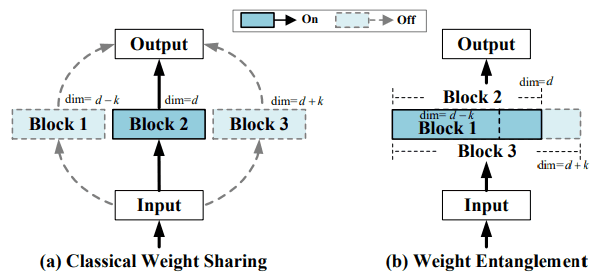In other words, AutoFormer selects the largest subset of blocks in the subnet that can be selected for exploration.

(The supernet will be identical to the largest architecture in the subnet.)

Here are the results of the comparison between the existing policy and Weight Entanglement.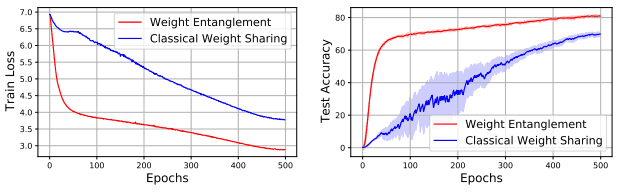The left figure shows the supernet train loss and the right figure shows the subnet ImageNet Top-1 Accuracy.

This ingenuity results in (1) faster convergence, (2) reduced memory costs, and (3) improved subnet performance.

AutoFormer performs an architectural search for three central search spaces (tiny/small/base). This is shown in the table below.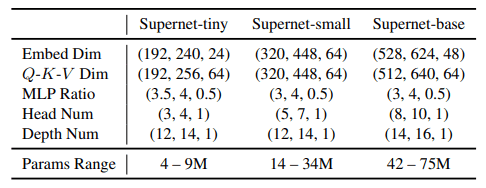The (a, b, c) in this table indicates (the lower limit, upper limit, and step) respectively. For example, (192,240,24) indicates that the search is performed for three-parameter settings of [192,216,240].

Similar to what I mentioned above about One-Shot NAS, AutoFormer's pipeline is divided into two stages.

During the training of the supernet, at each iteration, we randomly sample a subnet from the defined search space and update the corresponding weights of the supernet (the rest is frozen).

Phase 2: Evolutionary exploration of the architecture

To find the optimal architecture, we use an evolutionary algorithm to search for the subnet with the highest validation accuracy. Specifically, we follow the following steps

• Initially, we select $N$ random architectures as seeds.
• The top $k$ architectures are selected as parents, and the next generation is generated by Cross Over and Mutation.
• Crossing: Generates a new candidate based on two randomly selected candidates.
• Mutation: mutate the layer depth with probability $P_d$ and mutate each block with probability $P_m$ to generate a new candidate.

AutoFormer follows these pipelines to explore the optimal architecture.

## experimental results

In our experiments, we verify the performance of AutoFormer based on the following settings.

Supernets are trained the same way as DeiT, a Transformer-based method for visual tasks. The training settings, such as hyperparameters, are as follows.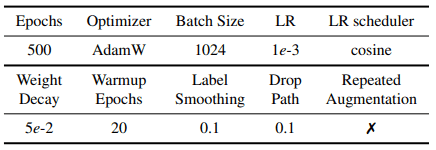For data augmentation, we use methods such as RandAugment, Cutmix, Mixup, and Random Erasing with the same settings as DeiT. The image is divided into 16x16 patches.

The evolutionary search follows the same protocol as SOPS. We use the validation set of ImageNet as the test set and 10,000 train examples as the validation set. The population size is set to 50, the number of generations is set to 20, the number of parental architectures is set to 10 for each generation, and the mutation probabilities are set to $P_d=0.2$ and $P_m=0.4$.

On Weight Entanglement and Evolutionary Search

First of all, the following are the results of validating the effectiveness of Weight Entanglement and Evolution Search (ESS) in AutoFormer.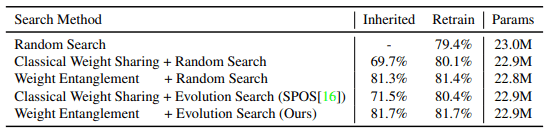In this table, Retrain shows the performance when the optimal architecture resulting from the search is retrained from scratch for 300 epochs (Inherited without retraining).

Surprisingly, when using Weight Entanglement, there is little change in performance between the two cases of no relearning and no relearning. A more detailed experiment on this point is shown below.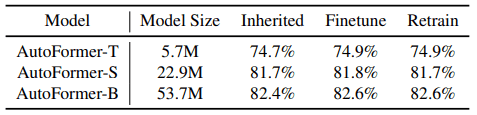As shown in the table, very little change in performance occurs with weights inherited from the supernet (Inherited), with 30 epochs of fine-tuning (Finetune), and with 300 epochs of relearning from scratch (Retrain).

The following figure also shows that many subnets perform well without relearning.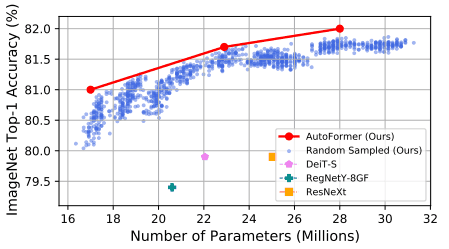These results show the effectiveness of using the performance of subnets whose weights are inherited from supernets as an index for comparing the superiority of architectures. In addition, the comparison results between random search and evolutionary search are as follows.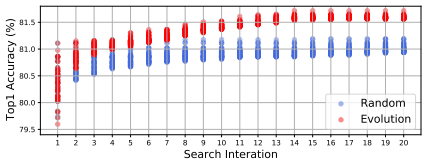In this figure, the performance of the top 50 for each generation is plotted, and we can see that evolutionary search is superior to random search.

### Comparison results with existing methods

The results of the comparison with AutoFormer on ImageNet for various existing CNN-based/Transformer-based methods are shown below.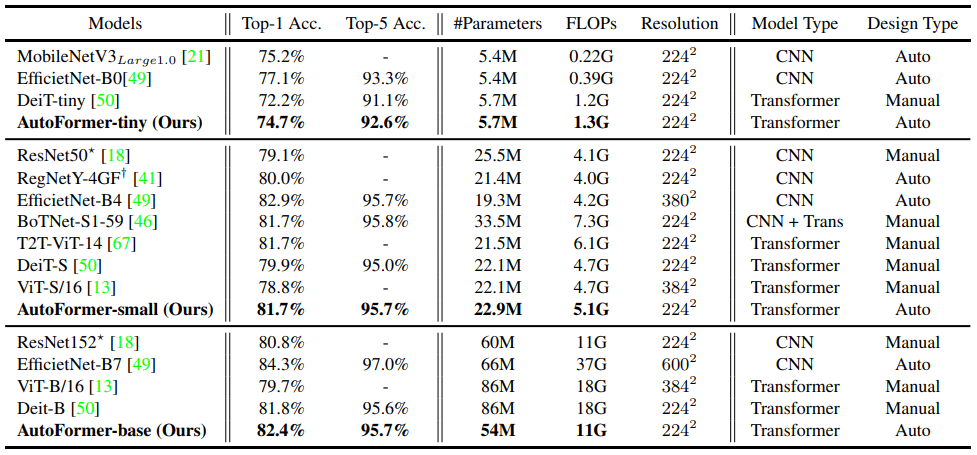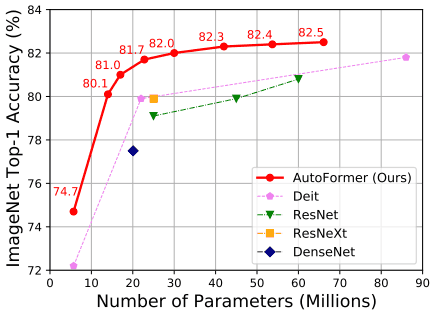The AutoFormer results show the results without relearning or fine-tuning and with the weights inherited from the supernet.

As shown in the table, the accuracy of our method is superior to that of the Transformer-based methods ViT and DeiT. It should be noted, however, that our method is still inferior to the CNN-based methods MobileNetV3 and EfficientNet, and does not outperform all existing methods for visual tasks.

The results of transfer training AutoFormer trained on ImageNet on a different dataset are shown below.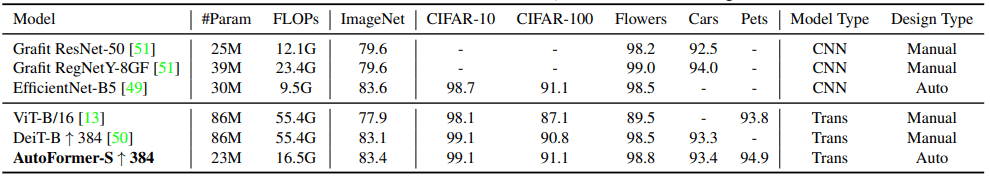In general, it can be seen that the accuracy is comparable to the existing methods even with a smaller number of parameters.